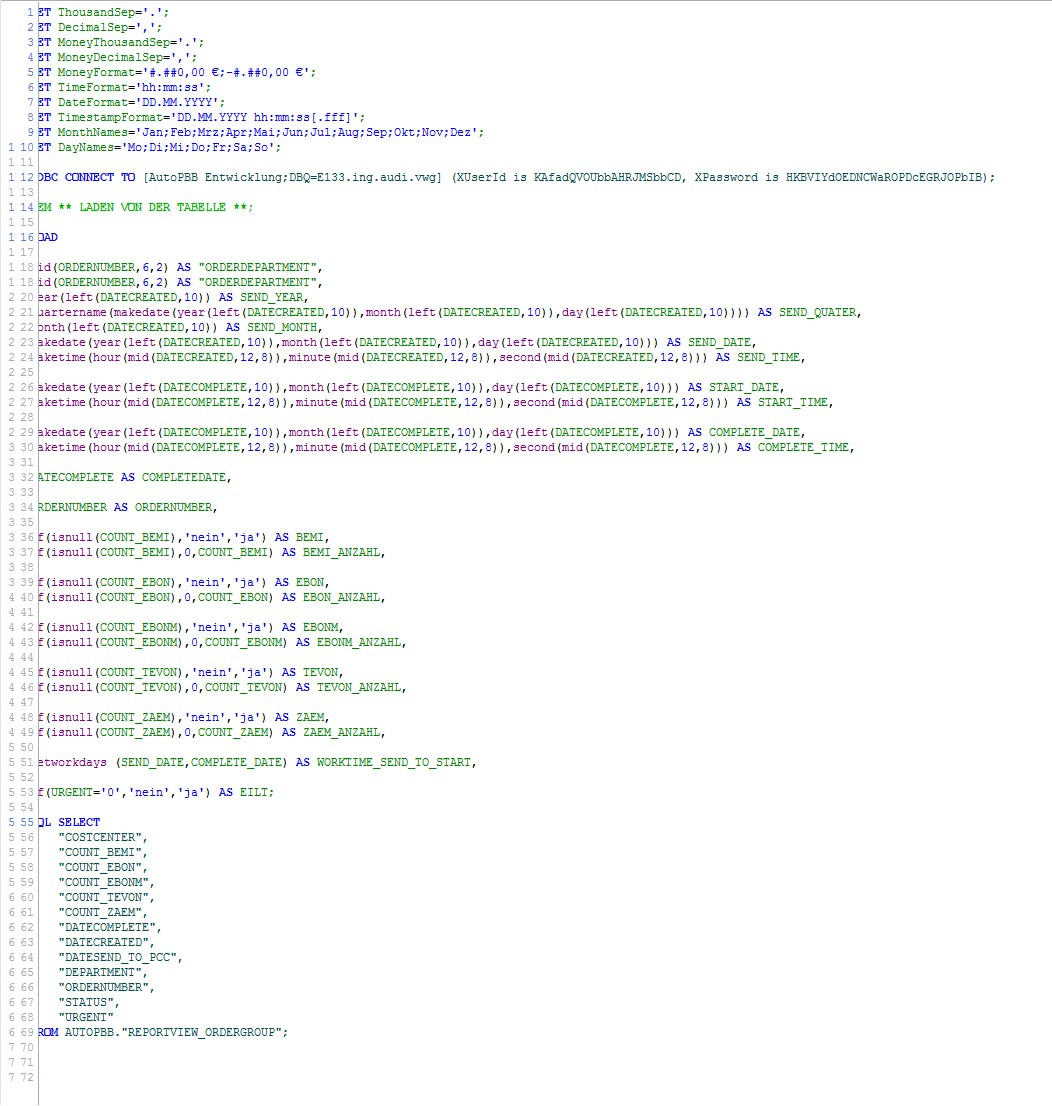# New to QlikView

Discussion board where members can get started with QlikView.

Announcements

#### Breathe easy -- you now have more time to plan your next steps with Qlik! QlikView 11.2 Extended Support is now valid through December 31, 2020. Click here for more information.Not applicable

## Use loaded data for calculations

hi, i'm new to qlikview and fighting with a little problem.

i'm loading data and transforming it with a formular and name it as value1, value2

afterwards i what to calculate a value 3 which is the difference between the two values.

value1

makedate(year(left(datecomplete,10)),month(left(datacomplete,10)),day(left(datecomplete,10))) AS VALUE1

value2

the same als value 1, just from a different field

if i'm using now the formulare

networkdays(value1,value2),

the script they no value 1

any ideas?

thanks for your help.

florian

Tags (3)
9 RepliesMVP

## Use loaded data for calculations

Hi,

Qlikview returns the correct answer.

Let me explain.

As you said you have Value1 and Value2 same as Value1.

So consider that your Value1 is 2011-01-01 so your value2 will be the same i.e 2011-01-01.

Now when you use Networkdays(Value1,Value2). If we put values in this expression, it becomes.

Networkdays(2011-01-01,2011-01-01)

So the result of the above expression is 1. As there is only one day in this.

Syntax of Networkdays()  is

networkdays ( start:date, end_date {, holiday} )

Hope you understood.

Regards,

Kaushik SolankiNot applicable

## Use loaded data for calculations

thanks for the answer, but the problem is a differnet one.

let me try to explain it again.

value 1 is always before value 2

the transromation process before is needed as the values which i get from the db is not usabel

value 1: 2011-01-01, value 2: 2011-01-03

value 3: should be 2

thanks for your helpMVP

## Use loaded data for calculations

Hi,

Can you please elobrate the problem as i am not clear with what ever you said.

Please use example if possible.

Regards,

Kaushik SolankiNot applicable

## Use loaded data for calculations

here a screenshot from the scriptNot applicable

## Re: Use loaded data for calculations

I am still somewhat new to qlikview, but try inserting your formulas

makedate(year(left(datecomplete,10)),month(left(datacomplete,10)),day(left(datecomplete,10)))

into your networkdays formula

IE:

Networkdays(makedate(year(left(datecomplete,10)),month(left(datacomplete,10)),day(left(datecomplete,10))),makedate(year(left(senddate,10)),month(left(senddate,10)),day(left(senddate,10))))

From what I have noticed about qlikview, if you define something earlier in the script, unless you set it to a variable it does not matter if it is later in the same load statement.

Message was edited by: marcslivingMVP

## Use loaded data for calculations

Hi,

The image is not that clear, and it is also cut from the left side.

One thing i saw from this image is that the date you are using in the networkdays are calcluated, right..?

if yes, then instead of using the name use the full calculation. Meaning something like this,

For example

Makedate(2011,01,05) as  Startdate,

Makedate(2011,01,01) as Enddate,

Networkdate(Makedate(2011,01,05),Makedate(2011,01,01)) as Difference

From xyz.

Regards,

Kaushik SolankiNot applicable

## Use loaded data for calculations

that would probably work, but as i've to make various calculations i don't want to work all the time with the whole expression. what do you meen with variable.Not applicable

## Use loaded data for calculations

What do you mean by work all the time with whole expression?

Networkdays(makedate(year(left(datecomplete,10)),month(left(datacomplete,10)),day(left(datecomplete,10))),makedate(year(left(senddate,10)),month(left(senddate,10)),day(left(senddate,10)))) as  datedifference

will set the number of days different as a field that you can use in the application.MVP & Luminary

## Re: Use loaded data for calculations

A couple suggestions. When you want to do calculations with a computed field, use preceding load to reference the new field and avoid repeating expressions. See http://qlikviewnotes.blogspot.com/2009/12/simplify-with-preceeding-load.html for more on preceding load.

Your use of MakeDate() MakeTime() in the script seems overly complex. It looks like you already have timestamp values in DATECOMPLETE and DATECREATED. So the difference is just:

DATECOMPLETE - DATECREATED

If you want to work with whole days only:

floor(DATECOMPLETE) - floor(DATECREATED)

-Rob

http://robwunderlich.com

Community Browser